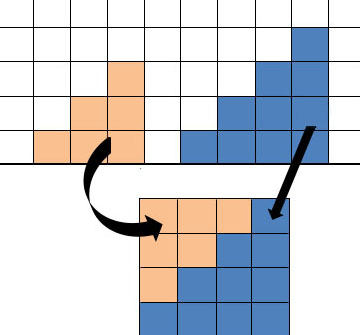# Triangular numbers

A teacher asked year 9 students to consider the following statement:

The sum of any two consecutive triangular numbers is a square number.

The teacher asked, "Is this true, and if so, can you prove it?"

The teacher observed and listened to students explaining their approaches as they worked and during a reporting back session. The teacher was able to identify various strategies and hence levels of reasoning.

• Trying particular cases
"Well, I thought of the triangular numbers, like 3, 6, 10. Three plus 6 equalled 9 and that is a square number. Six and 10 made 16, a square number. And I kept going like that. They all worked, so it is true."
• Trying extreme cases
"I tried a few examples and they worked, but that does not prove it is always true. I looked up bigger triangular numbers on the web and the ones I tried all worked, too. I tried –3 and –6 but that was +9. Maybe they do not all work, but I could not find any that didn't — they all made square numbers. So I reckon it’s true."
• Proof, using modelling
"I drew a triangular number, 6, like a right-angled triangle. Then I drew the next one, 10. I could see that the 6 and 10 would fit together like this, and make a square.Proof by modelling.

"Then I could see that it would always work, because no matter how many lines you have got, you have also got the smaller number that is one row less. Like 21 has 1 + 2 + 3 + 4 + 5 + 6, and the next triangular number down (15) is the same without the 6, so it will make a square. And that would be the case even with bigger numbers.
"Then I realised you could also draw it as an isosceles triangle and you could just put the smaller number underneath. I did not draw it but I can imagine it."
• Proof, using algebra
"First I developed a rule for the nth triangular number: multiply n by n + 1 then divide by 2, where n is the number of rows. Then I used it to write the rule for the (n + 1)th triangular number. I added these two rules together, then I used algebra to show that it is a square number. I found out something else, too: that the square number is the square of the difference between the two triangular numbers. I am working on a proof of that now."